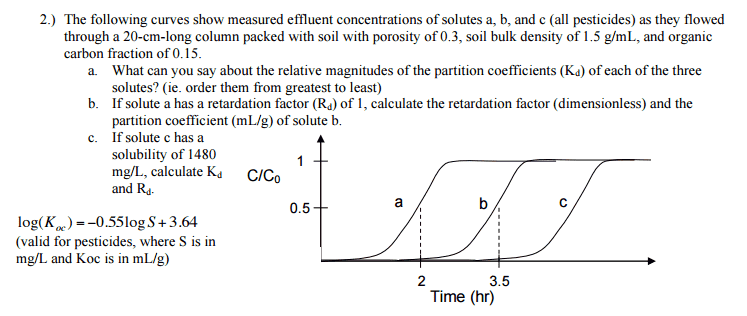# Partition Coefficient and Retardation Factor

• java22
The partition coefficient, Kd, is the fraction of a solute that is partitioned between the aqueous phase and the solid phase."

## Homework Statement## Homework Equations

Rd = retardation factor = (1 + (ƥbKd / n))

log(Koc) = -0.55logS + 3.64

Kd = Koc*foc

## The Attempt at a Solution

Part C is the only part I feel sure on. I simply plugged 1480 mg/L into the log(Koc) equation above to solve for Koc. Then I multiplied Koc by the foc given in the problem statement to obtain Kd. Finally, I plugged Kd in the Rd equation above along with the bulk density of the soil and the soil porosity given, and solved. Seems straight forward enough.

Part A is simply a concept-type question, but I do not know how to relate coefficients to this type of graph shown. Since there is no textbook for the class, I have no way to confirm if I'm right or not in my current thinking. I answered that the partition coefficient would be biggest for solute C and smallest for solute A, because a small partition coefficient would imply it dissolves in water more easily, shown by the graph for solute A reaching equilibrium the quickest. Am I correct here?

Part B is the oddest for me. At first I thought it was giving me the Rd of solute A so that I could plug in and solve for Kd, then use that Kd for the solute B to solve for its Rd. However, plugging in 1 for Rd gives a Kd of 0, so it must not be the right method. So, I simply compared the times it took solute A and solute B, and multiplied solute A's Rd by the same factor. So since 3.5 hours is 1.75x more than 2 hours, I did (1 * 1.75) = 1.75 for the Rd of solute B. I'm sure this method is totally wrong, but I have no references to find the right way, so any help would be much appreciated.

One thing that immediately let's me know I'm wrong somewhere is that I never used the 20 cm length given in the problem statement. Is this somehow used to find solute B's Rd?